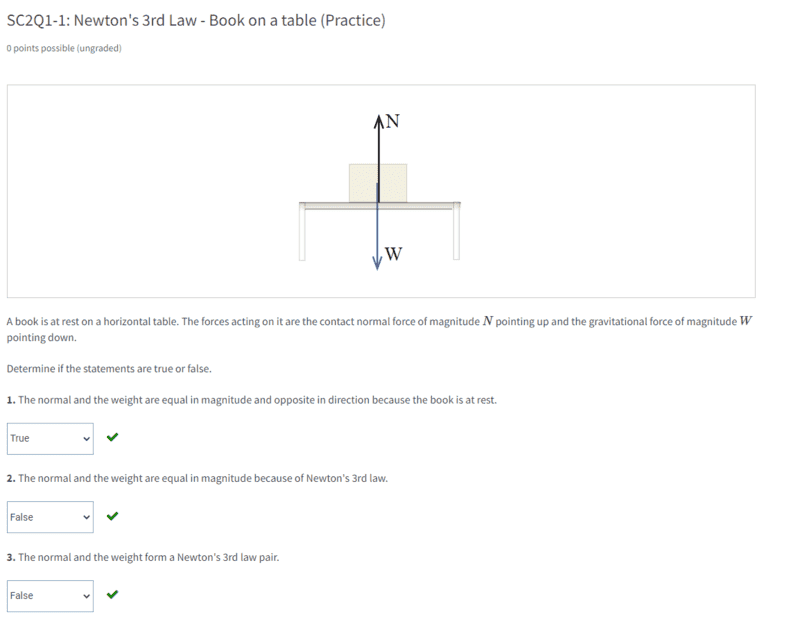# Newton's Third Law for book on a table

String theory guy
Homework Statement:
Statement is below.
Relevant Equations:
Newton's Third Law
This problem is from MIT's module again.I have a doubt about Newton's Third Law. How is the normal and weight of the book not Newton's 3rd Law pairs?

When the book is placed on the table, I imagine that the book's weight exerts a force on the table and the table exerts and equal and opposite force of the same magnitude and opposite direction. This force is called the normal force.

Gold Member
The Wikipedia entry for "Newton's Third Law" literally gives the answer to that specific question.

•String theory guy and topsquark
Gold Member
MHB
When the book is placed on the table, I imagine that the book's weight exerts a force on the table and the table exerts and equal and opposite force of the same magnitude and opposite direction. This force is called the normal force.
I'd write it out this way:
The book's weight, the force of the Earth's gravity acting on the book ##w_{BE}##, means that the book exerts a force on the table, ##N_{TB}##. Likewise, the table exerts an equal and oppositely directed force on the book, ##N_{BT}##. Third force law pairs are the same type of force, so one can't be a normal force and the other a weight, and they act between the two objects, Book and Table. So, the Third Law pairs are ##N_{BT}## and ##N_{TB}##.

The weight is the cause of the normal forces but is not the Third Law pair of either of them.

-Dan

••PeroK and String theory guy
Staff Emeritus
Homework Helper
Gold Member
The third law pairs are:
- The gravitational force from the Earth on the book and the gravitational force from the book on the Earth.
- The contact force from the table on the book and the contact force from the book on the table.

Third law pairs never affect the same object. The weight and the contact force on the book are equal and opposite because the book is in equilibrium, not because they are a third law pair. For example, if you add a force pushing down on the book, tilt or accelerate the table - they will not be the same any more. Third law pairs are always the force of A on B and the force of B on A.

•••Callumnc1, berkeman, PeroK and 2 others
String theory guy
I'd write it out this way:
The book's weight, the force of the Earth's gravity acting on the book ##w_{BE}##, means that the book exerts a force on the table, ##N_{TB}##. Likewise, the table exerts an equal and oppositely directed force on the book, ##N_{BT}##. Third force law pairs are the same type of force, so one can't be a normal force and the other a weight, and they act between the two objects, Book and Table. So, the Third Law pairs are ##N_{BT}## and ##N_{TB}##.

The weight is the cause of the normal forces but is not the Third Law pair of either of them.

-Dan
Thanks @topsquark. However, how dose the book exert a normal force onto the table? I don't understand why one can't be the weight and the other can't be the normal force from the table. The weight is equal to the normal force from the book onto the table.

•PeroK
Homework Helper
Gold Member
2022 Award
Thanks @topsquark. However, how dose the book exert a normal force onto the table? I don't understand why one can't be the weight and the other can't be the normal force from the table. The weight is equal to the normal force from the book onto the table.

••topsquark and String theory guy
Staff Emeritus
Homework Helper
Gold Member
However, how dose the book exert a normal force onto the table?
Because if the table acts on the book with a force ##\vec F##, then the book acts on the table with a force ##-\vec F##. That is what Newton's third law says.

••topsquark and String theory guy
••••